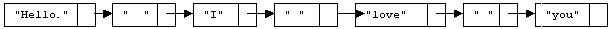# Text Justification Algorithm

### Purpose

```The Text Justification algorithm will ensure that the output from
your program is both left and right justified when displayed in a
mono-spaced font  such as Courier.  This  paragraph is an example
of such  justification.  All the  lines (except  the last) of the
output from  a given run  of your  program should  have the  same
length, and  the last  line is  to be  no longer  than the  other
lines.
```

## Overview

This pseudocode illustrates the Text Justification algorithm at a high level and how it should fit in to the Markov Chain programming assignment as a whole. The following sections describe the steps in more detail.

```build Markov Chain data structures;
while( more words to generate ) {
generate next word;
if( word is short enough to fit on current output line )
add word and trailing space(s) to the line;
// Two spaces if it is the end of a sentence.  See below.
else {
add spaces to justify the line; // details in phase 3
print the line;
add the word and trailing spaces to the line;
}
}
if( output line is not emtpy )
print output line;
```

## Algorithm Description

Construct a linked list that represents the current line of output. For each word in the output, add a node to the end of the list representing that word, then add another node to the end to signify the amount of space between that word and the next (initially, this is one space). You should also keep track of these space nodes in some type of array structure, to facilitate fast random access. When adding a new word would cause the combined length of all the words and spaces in the list to exceed the line length, stop. Remove the last node of the list (remember, this is the space from the last word on the line). Then distribute additional spaces among the space nodes (you can choose a reference randomly from the array you kept around and add a space to that node) until the combined length of the words and spaces in the list is equal to the prescribed line length. (Actually, you don't want all the space to be distributed randomly; see phase 3 below.) Traverse the list and output each word and set of spaces in order. Output a newline and start over. When you run out of words, output the remaining list without adding extra space, then terminate.

## Incremental Enhancement Plan

You are allowed to jump directly to Phase 3 if you wish, but the intermediate approaches may help you to get there.

Phase 1. Simply output one word per line. Or perhaps a fixed number (12 will probably work well) of words per line. Then you can debug the Markov Chain parts of your code before worrying about justifying the output. (If you get this far, and your Markov Chain algorithm is correct, you should earn about 60% of the correctness points for this assignment).

Phase 2. Don't output each word as you generate it. Instead, add it to a linked list of strings, one node for each word. Keep track of the total width of all of the words in your list (plus the spaces that will separate them when they are printed). When the width exceeds the output width, print all but the last word, and begin a new linked list containing just that last word. (If you get this far, and your Markov Chain algorithm is correct, you should earn about 80% of the correctness points for this problem).

Phase 3. Add (to your linked list of strings) nodes containing the spaces that separate the words (after you add a word to your list, you can add the space(s) that follow it). There will normally be one space, but if the word ends with a period, exclamation point, or question mark, there should be two spaces. For example, if the text so far is "Hello. I love you" The list will look like:Next, create an array (or ArrayList) of N references to the "space nodes" in the list (where N+1 is the number of words on this line). Calculate how many additional spaces (call this number M) must be added to the line in order to obtain a justified line.

• If M < N, randomly select M different array positions, and add one space to the string stored in each of the corresponding "space nodes".
• If M > N, add one space to each of the N space nodes, subtract N from M. Repeat this process until M < N or M = 0; then do the above M < N case or terminate, respectively.
• If M = N, add one space to each of the N space nodes, then terminate.

The reason for choosing random space nodes to enlarge is to avoid the appearance of more white space on one side of the text than on the other side, which would happen if you always added the spaces in the same places first. Notice that this approach avoids adding too many spaces in the same place; the number of added spaces in any two space nodes will either be the same or differ by one.

Once enough spaces have been added, traverse the list and print the strings, including the strings of spaces.

## Implementation

Obviously, you will need to utilize a linked list class to use this algorithm. The specifics of which library class to use are found in the main document.